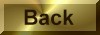Practice Problem 7

Use the values ofH° andS calculated in Practice Problem 5 to predict whether the following reaction is spontaneous at 25°C:

N2(g) + 3 H2(g)2 NH3(g)

Solution

According to Practie Problem 5, this reaction is favored by enthalpy but not by entropy:H° = -92.22 kJ    (favorable)S° = -198.75 J/K    (unfavorable)

Before we can compare these terms to see which is larger, we have to incorporate inot our calculation the tmeperature at which the reaction is run:

TK = 25o C + 273.15 = 298.15 K

We then multiply the entropy of reaction by the absolute temperature and subtract the TSo term from theHo term:Go =Ho - TSo

= -92,220 J - (298.15 K x -198.75 J/K)

= -92,220 J + 59,260 J

= -32,960 JGo = -32.96 kJ

According to this calculation, the reaction should be spontaneous at 25°C.